GFG App
Open AppBrowser
Continue

Algebraic division is the process of dividing a polynomial with another polynomial. It is the same as dividing a number by another number. There are two ways to divide polynomials. One of the most used ways to divide algebraic expressions is Algebraic Long Division.

Steps to perform Algebraic Long Division

1. Arrange the indices of a polynomial in descending order i.e., variables with higher exponents are arranged first followed by variables with lower exponents. Replace missing terms with 0. Example: 3x2+0x+1, 4x3+3x2+x-4
2. Divide the first term of dividend with the first term in divisor which gives the first term of quotient.
3. Perform multiplication between divisor and the first term of quotient.
4. Subtract the result obtained in step-3 from the dividend and bring down the next term. This will be our new dividend.
5. Repeat step-2 to step-4 to find the next term of quotient.
6. This process is continued until we get the remainder. This may be zero or index less than the divisor.

### Sample Questions

Question 1: Perform algebraic long division (6x2+7x-20)÷(2x+5)

Solution: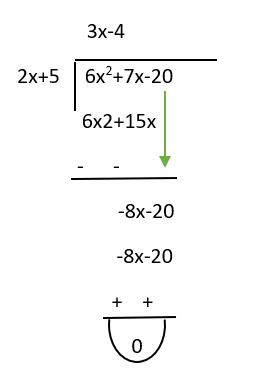Quotient=3x-4

Remainder=0

Question 2: Perform algebraic long division between dividend 2x3-3x2-3x+2 and divisor x-2

Solution: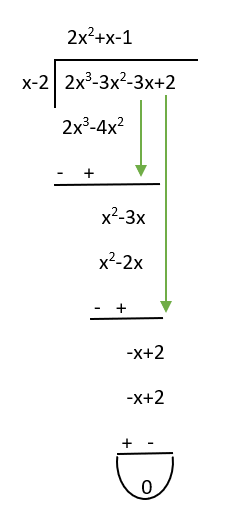Quotient=2x2+x-1

Remainder=0

Question 3: (3x4+x3-17x2+19x-6)÷(x2-2x+1) (Use Algebraic long division method)

Solution: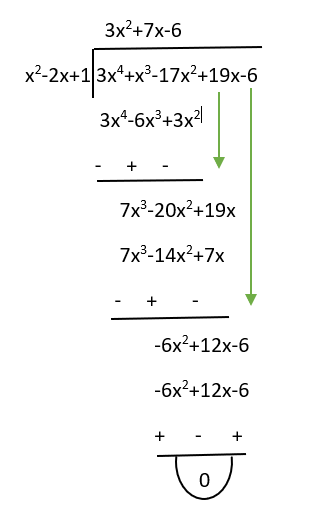Quotient=3x2+7x-6

Remainder=0

Question 4: Solve (2x2+7x-4)÷(x-4) using long division approach

Solution: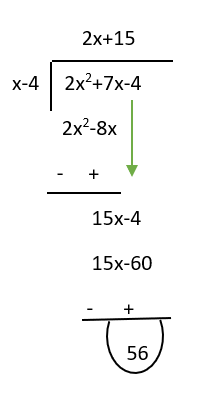Here we got remainder 56 indicates that given divisor is not the factor of dividend.

So, (2x2+7x-4)÷(x-4)=2x+15+(56/x-4)

Question 5: Solve (x3-12x2-20)÷(x2-2x+1) using algebraic long division

Solution: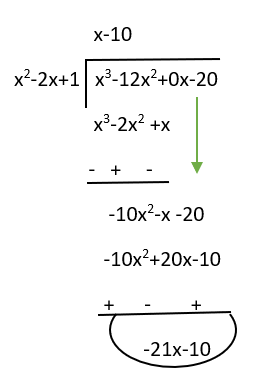Here the remainder is -21x-10 indicates that given divisor is not the factor of dividend.

So, (x3-12x2-20)÷(x2-2x+1)=x-10+((-21x-10)/x2-2x+1))

My Personal Notes arrow_drop_up